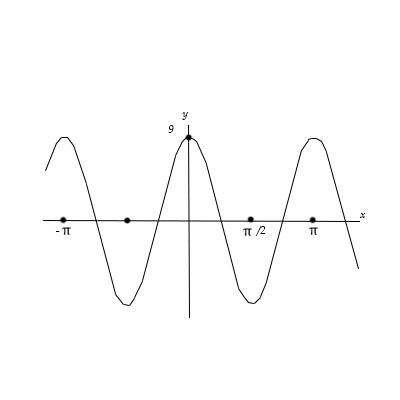# The graph of a sine function with a positive coefficient is shown below. a. Find the amplitude,...

## Question:

The graph of a sine function with a positive coefficient is shown below.

a. Find the amplitude, period and phase shift.

b. Write the equation in the form {eq}y=a \sin (bx-c) {/eq} for {eq}a>0,b>0 {/eq}, and the least positive real number {eq}c {/eq}.## Sine Function:

The sine function is a function defined by the following formula

{eq}y=a \sin (bx-c) {/eq}

in which a is the amplitude, {eq}2\pi/b {/eq} is the period and c is the phase shift.

Consider a sine function in the form

{eq}y=a \sin(bx-c) {/eq}

where a is the amplitude, {eq}\frac{2\pi}{b} {/eq} is the period and {eq}c {/eq} is the phase shift.

Based on the graph of the sine function given as input data,

we see that the amplitude is

{eq}a=9, {/eq}

the period is

{eq}\displaystyle \frac{2\pi}{b} = \pi \Rightarrow b = 2 {/eq}

and the least positive phase shift is

{eq}\displaystyle c=\frac{3\pi}{2}. {/eq}

Therefore, the sine function is written as

{eq}y=9 \sin(2x-\frac{3\pi}{2} ). {/eq}